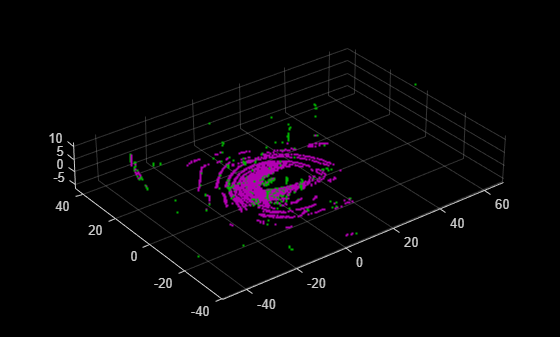# findPose

Find absolute pose of points in map

Since R2022b

## Syntax

``absPose = findPose(loamMap,points,relPose)``
``absPose = findPose(___,localMapSize)``
``[absPose,optimizedRelPose] = findPose(___)``
``[absPose,optimizedRelPose,rmse] = findPose(___)``
``[___] = findPose(___,Name=Value)``

## Description

example

````absPose = findPose(loamMap,points,relPose)` returns the optimized absolute pose that aligns the specified points to the points in the lidar odometry and mapping (LOAM) map `loamMap`.```
````absPose = findPose(___,localMapSize)` specifies the size of the local map used to refine the absolute pose, in addition to all input arguments from the previous syntax. When you do not specify `localMapSize`, the function uses a map size defined by the x, y, and z spatial extents of all the input points enlarged on all sides by a spatial radius, `SearchRadius`, centered around the estimated absolute pose `absPose`.```
````[absPose,optimizedRelPose] = findPose(___)` returns the optimized relative pose.```
````[absPose,optimizedRelPose,rmse] = findPose(___)` returns the root mean squared error of the Euclidean distance between the aligned points. Lower values indicate a more accurate alignment.```
````[___] = findPose(___,Name=Value)` specifies options using one or more name-value arguments in addition to any combination of arguments from previous syntaxes. For example, `SearchRadius=4` sets the search radius for point matching to `4`.```

## Examples

collapse all

Create a map to store LOAM feature points.

```voxelSize = 0.1; loamMap = pcmaploam(voxelSize);```

Create a `velodyneFileReader` object to read point cloud daata.

`veloReader = velodyneFileReader("lidarData_ConstructionRoad.pcap","HDL32E");`

Read the first point cloud into the workspace.

`ptCloud1 = readFrame(veloReader,1);`

Detect LOAM feature points.

`points1 = detectLOAMFeatures(ptCloud1);`

Downsample the less planar surface points to improve registration speed.

```gridStep = 1; points1 = downsampleLessPlanar(points1,gridStep);```

Add the LOAM points of the first point cloud to the map.

```absPose = rigidtform3d; addPoints(loamMap,points1,absPose)```

Read the fifth point cloud, and detect the LOAM feature points in it.

```ptCloud2 = readFrame(veloReader,5); points2 = detectLOAMFeatures(ptCloud2);```

Downsample the less planar surface points.

`points2 = downsampleLessPlanar(points2,gridStep);`

Get a relative pose estimate by using the `pcregisterloam` function.

`relPose = pcregisterloam(points2,points1);`

Find the absolute pose of the points from the fifth point cloud in the map.

`absPose = findPose(loamMap,points2,relPose);`

Add the points from the fifth point cloud to the map.

`addPoints(loamMap,points2,absPose)`

Visualize the map.

```figure show(loamMap,MarkerSize=20) ```## Input Arguments

collapse all

LOAM map, specified as a `pcmaploam` object.

LOAM points, specified as a `LOAMPoints` object.

Relative pose, specified as a `rigidtform3d` object. The `relPose` input contains the relative pose between the new input points and the last points added to the LOAM map `loamMap` in the sensor frame. You can use the `pcregisterloam` function to estimate the relative pose.

Local map size, specified as a three-element vector of the form [dx dy dz]. When you do not specify `localMapSize`, the function uses a map size defined by the x, y, and z spatial extents of all the input points enlarged on all sides by a spatial radius, `SearchRadius`, centered around the estimated absolute pose `absPose`.

### Name-Value Arguments

Specify optional pairs of arguments as `Name1=Value1,...,NameN=ValueN`, where `Name` is the argument name and `Value` is the corresponding value. Name-value arguments must appear after other arguments, but the order of the pairs does not matter.

Example: `findPose(loamMap,points,relPose,SearchRadius=4)` sets the search radius for point matching to `4`.

Search radius for point matching, specified as a positive integer. When matching, the function finds the closest edge and surface points within the specified radius. For best results, set this value based on the certainty of the relative pose, specified by the `relPose` input. You can increase the value of `SearchRadius` when there is greater uncertainty in the relative pose input, but this can also decrease the registration speed.

Maximum iterations before LOAM registration stops, specified as a positive integer.

Tolerance between consecutive LOAM iterations, specified as a two-element vector, with nonnegative values, of the form [Tdiff Rdiff].

• Tdiff — Tolerance for the estimated absolute difference in translation and rotation between consecutive LOAM iterations. Measures the Euclidean distance between two translation vectors.

• Rdiff — Tolerance for the estimated absolute difference of the angular rotation between consecutive iterations, measured in degrees.

The algorithm stops when the difference between the estimates of the rigid transformations in the most recent consecutive iteration falls below the specified tolerance value.

Display progress information, specified as a numeric or logical `0` (`false`) or `1` (`true`). To display progress information, set `Verbose` to `true`.

## Output Arguments

collapse all

Absolute pose for aligning new points to the existing points in a LOAM map, returned as a `rigidtform3d` object. The `addPoints` function uses the absolute pose to align new points to the existing map.

Optimized relative pose, returned as a `rigidtform3d` object.

Root mean squared error, returned as a positive scalar that described the Euclidean distance between the aligned points. Lower values indicate a more accurate alignment.

## Version History

Introduced in R2022b